# Math in Focus Grade 4 Cumulative Review Chapters 7 and 8 Answer Key

Practice the problems of Math in Focus Grade 4 Workbook Answer Key Cumulative Review Chapters 7 and 8 to score better marks in the exam.

## Math in Focus Grade 4 Cumulative Review Chapters 7 and 8 Answer Key

Concepts and Skills

write each fraction or mixed number as a decimal. (Lesson 7.1)

Question 1.
$$\frac{4}{10}$$ = ____
$$\frac{4}{10}$$ =  0.4.

Explanation:
$$\frac{4}{10}$$ = 4 ÷ 10 = 2 ÷ 5 = 0.4.

Question 2.
3$$\frac{3}{10}$$ = ____
3$$\frac{3}{10}$$ = 0.9.

Explanation:
3$$\frac{3}{10}$$ = 3(3 ÷ 10) = 3(0.3) = 0.9.

Question 3.
$$\frac{18}{10}$$ = ____
$$\frac{18}{10}$$ = 1.8.

Explanation:
$$\frac{18}{10}$$ = 18 ÷ 10 = 9÷ 5 = 1.8.

Write each decimal in tenths. (Lesson 7.1)
Question 4.
0.6 = ___ tenths
0.6 = 6 tenths.

Explanation: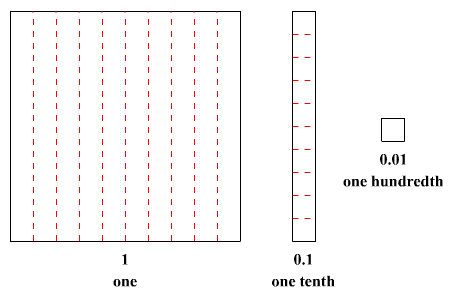0.6 = 6 tenths.

Question 5.
1.7 = ___ tenths
1.7 = 17 tenths.

Explanation:
1.7 = 17 tenths.

Question 6.
9.5 = ___ tenths
9.5 = 95 tenths.

Explanation:
9.5 = 95 tenths.

Question 7.
4.2 = ___ tenths
4.2 = 42 tenths.

Explanation:
4.2 = 42 tenths.

write each of these as a decimal. (Lesson 7.1)
Question 8.
3 ones and 4 tenths = ___
3 ones and 4 tenths = 3.4.

Explanation:
3 ones and 4 tenths = 3.4.

Question 9.
8 ones and 1 tenth = ____
8 ones and 1 tenth = 8.1.

Explanation:
8 ones and 1 tenth = 8.1.

Question 10.
77 tenths = ___
77 tenths = 7.7.

Explanation:
77 tenths = 7.7.

Question 11.
19 tenths = ____
19 tenths = 1.9.

Explanation:
19 tenths = 1.9.

Fill in the blanks. (Lesson 7.1)
Question 12.
22 tenths = 2 ones and ___ tenths
22 tenths = 2 ones and  2 tenths.

Explanation:
22 tenths = 2 ones and 2 tenths.

Question 13.
3.2 = 3 ones and ___ tenths
3.2 = 3 ones and 2 tenths.

Explanation:
3.2 = 3 ones and 2 tenths.

Write the correct decimal in each box. (Lesson 7.1)
Question 14.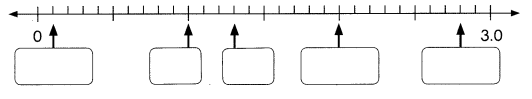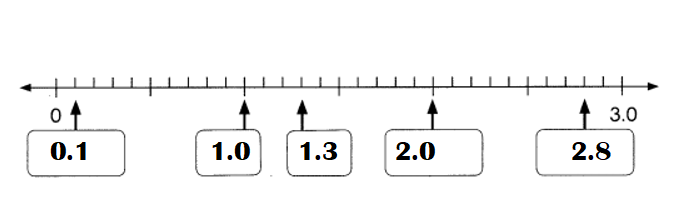Explanation:
1. 1 tenths.
2.1 one.
3. 1 one 3 tenths.
4. 2 one o tenths.
5. 2 one 8 tenths.Complete the expanded form of each decimal. (Lesson 7.1)
Question 15.
5.4 = 5 + ___
5.4 = 5 + 4 tenths.

Explanation:
5.4 = 5 + 4 tenths.

Question 16.
7.1 = 7 + ___
7.1 = 7 + 1 tenths.

Explanation:
7.1 = 7 + 1 tenths.

Question 17.
3.6 = 3 + __.
3.6 = 3 + 6 tenths.

Explanation:
3.6 = 3 + 6 tenths.

Question 18.
10.2 = 10 + ___
10.2 = 10 + 2 tenths.

Explanation:
10.2 = 10 + 2 tenths.

Fill in the blanks. (Lesson 7.1)
Question 19.
In 22.3, the digit 3 is in the ___ place. Its value is ____.
In 22.3, the digit 3 is in the tenths place. Its value is 3 ÷ 10.

Explanation:
In 22.3,
the digit 3 is in the tenths place.
Its value is $$\frac{3}{10}$$ = 3 ÷ 10.

Write each fraction or mixed number as a decimal. (Lesson 7.2)
Question 20.
$$\frac{9}{100}$$ = ____
$$\frac{9}{100}$$ = 0.09.

Explanation:
$$\frac{9}{100}$$ = 9 ÷ 100 = 0.09.

Question 21.
2$$\frac{26}{100}$$ = ____
2$$\frac{26}{100}$$ = 0.52.

Explanation:
2$$\frac{26}{100}$$ = 2(26 ÷ 100)
= 2 × 0.26
= 0.52.

Question 3.
$$\frac{105}{100}$$ = ____
$$\frac{105}{100}$$ = 1.05.

Explanation:
$$\frac{105}{100}$$ = 105 ÷ 100 = 1.05.

Write each decimal in hundredths. (Lesson 7.2)
Question 23.
0.06 = ___ hundredths
0.06 = 6 hundredths.

Explanation:
0.06 = 6 hundredths.

Question 24.
1.33 = ___ hundredths
1.33 = 133 hundredths.

Explanation:
1.33 = 133 hundredths.

Question 25.
2.5 = ___ hundredths
2.5 = 250 hundredths.

Explanation:
2.5 = 250 hundredths.

Write each of these as a decimal. (Lesson 7.2)
Question 26.
2 ones and 6 hundredths = ____
2 ones and 6 hundredths = 2.06.

Explanation:
2 ones and 6 hundredths = 2.06.

Question 27.
5 tenths 5 hundredths = ____
5 tenths 5 hundredths = 0.55.

Explanation:
5 tenths 5 hundredths = 0.55.

Question 28.
7 ones and 3 tenths 4 hundredths = ___
7 ones and 3 tenths 4 hundredths = 7.34

Explanation:
7 ones and 3 tenths 4 hundredths = 7.34.

Fill in the blanks. (Lesson 7.2)
Question 29.
16 hundredths = 1 tenth ___ hundredths
16 hundredths = 1 tenth 6 hundredths.

Explanation:
16 hundredths = 1 tenth 6 hundredths.

Question 30.
0.45 = 4 tenths ___ hundredths
0.45 = 4 tenths 5 hundredths.

Explanation:
0.45 = 4 tenths 5 hundredths.

Mark ✗ to show where each decimal is located on the number line. Label its value. (Lesson 7.2)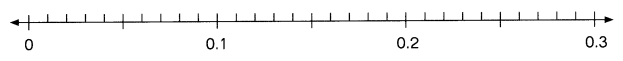Question 31.
0.04
0.04 = 4 hundredths.

Explanation: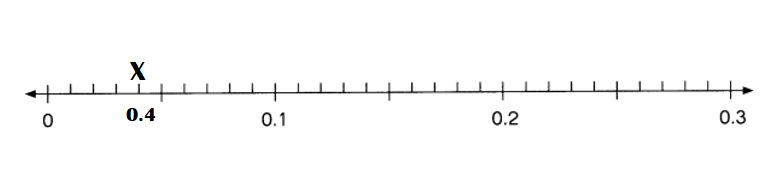Question 32.
0.15
0.15 = 0 ones 1 tenths 5 hundredths.

Explanation: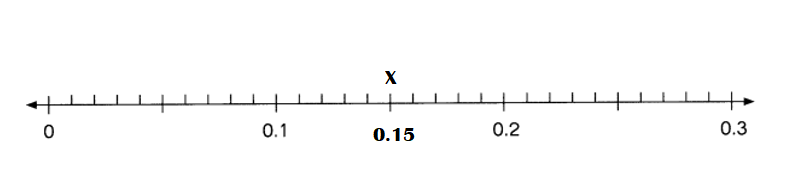Question 33.
0.26
0.26 = 0 ones 2 tenths 6 hundredths.

Explanation: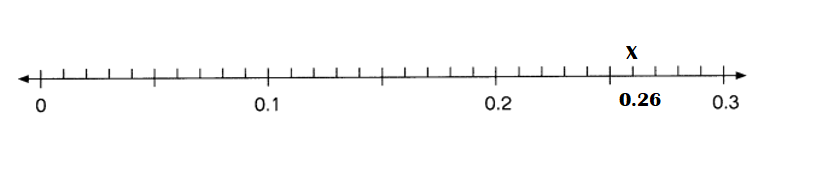Complete.
Question 34.
5.2 = __________ ones and __________ tenths
5.2 = 5 ones and 2 tenths.

Explanation:
5.2 = 5 ones and 2 tenths.

Question 35.
0.86 = ___________ tenths ___________ hundredths
0.86 = 8 tenths 6 hundredths.

Explanation:
0.86 = 8 tenths 6 hundredths.

Question 36.
3.7 = ___________ tenths
3.7 = 7 tenths.

Explanation:
3.7 = 7 tenths.

Question 37.
0.93 = __________ hundredths
0.93 = 3 hundredths.

Explanation:
0.93 = 3 hundredths.

Write each sum as a decimal. (Lesson 7.2)
Question 38.
7 + 0.6 + 0.02 = ___
7 + 0.6 + 0.02 = 7.62.

Explanation:
7 + 0.6 + 0.02 = 7.6 + 0.02
= 7.62.

Question 39.
10 + 0.4 + 0.04 = ___
10 + 0.4 + 0.04 = 10.44.

Explanation:
10 + 0.4 + 0.04 =  10.4 + 0.04
= 10.44.

Question 40.
5 + $$\frac{1}{10}$$ + $$\frac{8}{100}$$ = ___
5 + $$\frac{1}{10}$$ + $$\frac{8}{100}$$ = 5.09.

Explanation:
5 + $$\frac{1}{10}$$ + $$\frac{8}{100}$$ = 5 + (1 ÷ 100) + (8 ÷ 100)
= 5 + 0.1 + 0.08
= 5.1 + 0.08
= 5.18.

Question 41.
9 + $$\frac{3}{10}$$ + $$\frac{7}{100}$$ = ___
9 + $$\frac{3}{10}$$ + $$\frac{7}{100}$$ = 9.37.

Explanation:
9 + $$\frac{3}{10}$$ + $$\frac{7}{100}$$ = 9 + (3 ÷ 10) + (7 ÷ 100)
= 9 + 0.3 + 0.07
= 9.3 + 0.07
= 9.37.

Fill in the blanks. (Lesson 7.2)
Question 42.
In 14.68, the digit 8 is in the ___ place. Its value is ___.
In 14.68, the digit 8 is in the hundredths place. Its value is 8 hundredths.

Explanation:
In 14.68,
the digit 8 is in the hundredths place. Its value is 8 hundredths.

Fill in the blanks. (Lesson 7.2)
Question 43.
$0.75 = ___ cents Answer:$0.75 = 75 cents.

Explanation:
Conversion: 1 dollar = 100 cents.
$0.75 = 100 × 0.75 = 75 cents. Question 44.$12.25 = ___ cents
$12.25 = 1225 cents. Explanation: Conversion: 1 dollar = 100 cents.$12.25 = 100 × 12.25
= 1225 cents.

Question 45.
$8.05 = ___ cents Answer:$8.05 = 805 cents.

Explanation:
Conversion: 1 dollar = 100 cents.
$8.05 = 100 × 8.05 = 805 cents. Write each amount of money in decimal form. (Lesson 7.2) Question 46. 65 cents =$___
65 cents = $___ Explanation: Conversion: 1 dollar = 100 cents. 65 cents = 65 ÷ 100 =$ 0.65.

Question 47.
10 dollars and 90 cents = $___ Answer: 10 dollars and 90 cents =$10.90.

Explanation:
Conversion: 1 dollar = 100 cents.
10 dollars and 90 cents = 10 + (90 ÷ 100)
= 10 + 0.90
= $10.90. Question 48. 2 dollars and 5 cents =$____
2 dollars and 5 cents = $2.05. Explanation: Conversion: 1 dollar = 100 cents. 2 dollars and 5 cents = 2 + (5 ÷ 100) = 2 + 0.05 =$2.05.

Fill in the blanks. (Lesson 7.3)
Question 49.
0.1 more than 1.1 is ____
0.1 more than 1.1 =  1.2.

Explanation:
0.1 more than 1.1 =  0.1 + 1.1 = 1.2.

Question 50.
0.2 less than 2 is ____
0.2 less than 2 = 0.8.

Explanation:
0.2 less than 2 =  2 – 0.2 = 0.8.

Question 51.
0.01 less than 0.1 is ___
0.01 less than 0.1 = 0.09.

Explanation:
0.01 less than 0.1 =  0.1 – 0.01 = 0.09.

Question 52.
0.03 more than 0.07 is ___
0.03 more than 0.07 = 0.10.

Explanation:
0.03 more than 0.07 =  0.07 + 0.03 = 0.10.

Mark ✗ to show where each decimal is located on the number line. Label its value. (Lesson 7.3)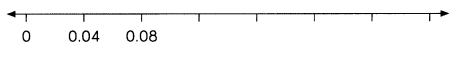Question 53.
0.16
0.16 = 0 ones 1 tenths 6 hundredths.

Explanation: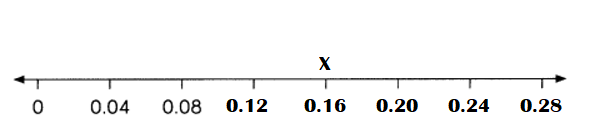Question 54.
0.24
0.24 = 0 ones 1 tenths 6 hundredths.

Explanation: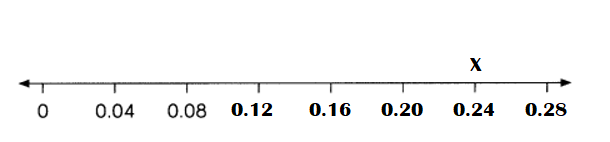Compare. Write > or <. (Lesson 7.3)
Question 55.
4.1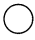0.41
4.1 > 0.41.

Explanation:
4.1 is greater than 0.41 as its ones place number is greater in comparison.

Question 56.
0.730.70
0.73 < 0.70.

Explanation:
0.73  is lesser than 0.70 as its hundredths place number in comparison comes first in comparison.

Circle the greatest decimal and underline the least. (Lesson 7.3)
Question 57.
3.04 3.4 0.34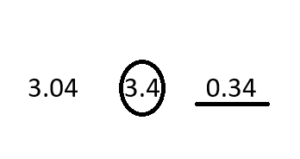Explanation:
3.4 is comparatively greater than 3.04 and 0.34 as its tenths place number is greater.
0.34 is comparatively lesser than the 3.4 and 3.04 as its ones place number is less.

Question 58.
0.6 0.61 0.65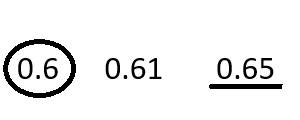Explanation:
0.6 is comparatively greater than 0.61 and 0.65 as its tenths place number comes first in number line.
0.65 is comparatively lesser than the 0.6 and 0.61 as its comes last in number line.

Fill in the blank. (Lesson 7.3)
Question 59.
Write a decimal that is greater than 0.9 but less than 1.0. ____
A decimal that is greater than 0.9 but less than 1.0 is 0.987.

Explanation:
A decimal that is greater than 0.9 but less than 1.0 is 0.987.
The largest nearest number that can be approximated to 0.9 is 0.94999999999.
The lowest nearest number that can be approx. to 0.987 is 0.986500000000000000000
Hence, 0.987 is larger than 0.9.
Such problems are categorized under “ Number Theory”.

Round each decimal to the nearest whole number. (Lesson 7.4)
Question 60.
4.36 = ___
4.36 = 4.0.

Explanation:
Rounding 4.36 to its nearest whole number = 4.0.

Question 61.
7.81 = ___
7.81 = 8.0.

Explanation:
Rounding 7.81  to its nearest whole number = 8.0.

Question 62.
5.07 = ___
5.07 = 5.0.

Explanation:
Rounding 5.07 to its nearest whole number = 5.0.

Round each decimal to the nearest tenth. (Lesson 7.4)
Question 63.
2.39 = ___
2.39 = 2.50.

Explanation:
Rounding 2.39 to its nearest tenth = 2.50.

Question 64.
6.63 = ___
6.63 = 7.0.

Explanation:
Rounding 6.63 to its nearest tenth = 7.0.

Question 65.
4.00 = ___
4.00 = 4.0.

Explanation:
Rounding 4.00 to its nearest tenth = 4.0.

write each decimal as a fraction in simplest form. (Lesson 7.5)
Question 66.
0.6 =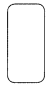0.6 =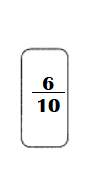Explanation:
simplest form of 0.6 = 6 ÷10.

Question 67.
0.55 =0.55 =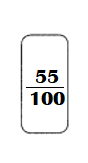Explanation:
simplest form of  0.55 = 55 ÷ 100.

write each fraction or mixed number as a decimal. (Lesson 7.5)
Question 68.
$$\frac{1}{5}$$ =
$$\frac{1}{5}$$ = 0.2.

Explanation:
$$\frac{1}{5}$$ = 1 ÷ 5 = 0.2.

Question 69.
$$\frac{9}{20}$$ =
$$\frac{9}{20}$$ = 0.45.

Explanation:
$$\frac{9}{20}$$ = 9 ÷ 20 = 0.45.

Question 70.
$$\frac{5}{2}$$ =
$$\frac{5}{2}$$ = 2.50.

Explanation:
$$\frac{5}{2}$$ = 5 ÷ 2 = 2.50.

Question 71.
1$$\frac{3}{4}$$ =
1$$\frac{3}{4}$$ = 1.75.

Explanation:
1$$\frac{3}{4}$$ = 1 (3 ÷ 4)
= (4 + 3) ÷ 4
= 7 ÷ 4
= 1.75.

Question 72.
4$$\frac{2}{5}$$ =
4$$\frac{2}{5}$$ = 4.4.

Explanation:
4$$\frac{2}{5}$$ = 4 (2 ÷ 5)
= (20+2) ÷ 5
= 22 ÷ 5
= 4.4.

Question 73.
5$$\frac{1}{4}$$ =
5$$\frac{1}{4}$$ = 5.25.

Explanation:
5$$\frac{1}{4}$$ = 5 (1 ÷ 4)
= (20 + 1) ÷ 4
= 21 ÷ 4
= 5.25.

Find each sum or difference. (Lesson 8.1 and 8.2)

Question 74.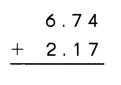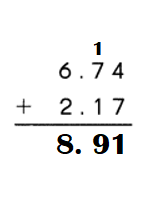Explanation:
6.74 + 2.17 = 8.91.

Question 75.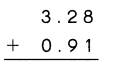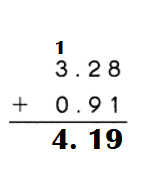Explanation:
3.28 + 0.91 = 4.19.

Question 76.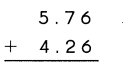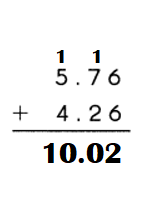Explanation:
5.76 + 4.26 = 10.02.

Question 77.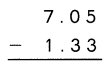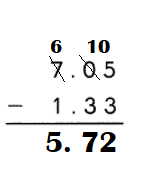Explanation:
7.05 – 1.33 = 5.72.

Question 78.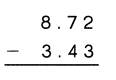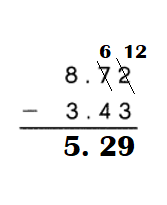Explanation:
8.72 – 3.43 = 5.29.

Question 79.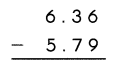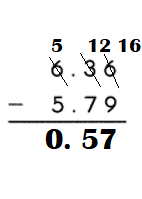Explanation:
6.36 – 5.79 = 0.57.

Problem Solving
Solve. Show your work. (Lesson 8.3)
Question 80.
Lina thinks of a number. When she adds 9.65 to it, she gets 20.7. What number is Lina thinking of?
The number Lina thinks = 11.05.

Explanation:
When she adds 9.65 to it, she gets 20.7.
Let the number be X.
=> X + 9.65 = 20.7.
=> X = 20.7 – 9.65
=> X = 11.05.

Question 81.
Suri bought a skirt for $25.90 and a sweatshirt for$19.90. She paid the cashier $50. How much change did she receive? Answer: Amount of change she received =$4.2.

Explanation:
Cost of a skirt Suri bought = $25.90. Cost of a sweatshirt Suri bought =$19.90.
Amount of money she paid to the cashier = $50. Amount of change she received = Amount of money she paid to the cashier – (Cost of a skirt Suri bought + Cost of a sweatshirt Suri bought) =$50 – ($25.90 +$19.90)
= $50 –$45.8
= \$4.2.